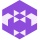Home
IT Knowledge
Inspiration
Languages
EN

# Python - arithmetic operators

0 points
Created by:Dale-K1
327

In this article, we would like to show you arithmetic operators in Python.

## Arithmetic operators

OperatorExampleName
-a - bsubtraction
*a * bmultiplication
/a / bdivision
%a % bmodulus
**a ** bexponentiation
//a // bfloor division

## Practical example

``````a = 3
b = 2

# results
print(a + b)   # 5
print(a - b)   # 1
print(a * b)   # 6
print(a / b)   # 1.5
print(a % b)   # 1
print(a ** b)  # 9
print(a // b)  # 1``````

Output:

``````5
1
6
1.5
1
9
1``````